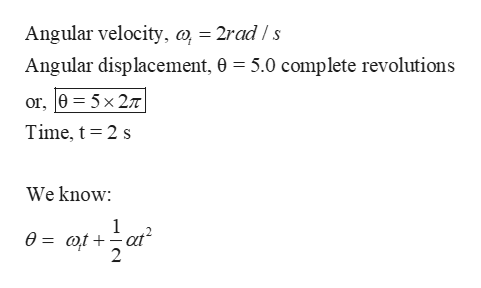# At t = 0, a wheel rotating about a fixed axis at a constant angular acceleration has an angular velocity of 2.0 rad/s. Two seconds later it has turned through 5.0 complete revolutions. What is the angular acceleration of this wheel, in units of rad/s2?

Question
1 views

At t = 0, a wheel rotating about a fixed axis at a constant angular acceleration has an angular velocity of 2.0 rad/s. Two seconds later it has turned through 5.0 complete revolutions. What is the angular acceleration of this wheel, in units of rad/s2?

check_circle

Step 1

The expression for angular displacement, will be :help_outlineImage TranscriptioncloseAngular velocity, m, = 2rad / s Angular displacement, 0 = 5.0 complete revolutions or, 0 = 5x 27T Time, t= 2 s We know: .2 at? 0 = @t+ 2 fullscreen
Step 2

Re-arrange the expression to find the an...

### Want to see the full answer?

See Solution

#### Want to see this answer and more?

Solutions are written by subject experts who are available 24/7. Questions are typically answered within 1 hour.*

See Solution
*Response times may vary by subject and question.
Tagged in

### Angular Motion